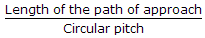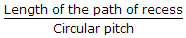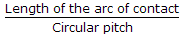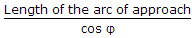# Mechanical Engineering - Theory of machines

### Exercise :: Theory of machines - Section 10

1.

The pitching of a ship produces forces on the bearings which act __________ to the motion of the ship.

 A. vertically and parallel B. vertically and perpendicular C. horizontally and parallel D. horizontally and perpendicular

Explanation:

No answer description available for this question. Let us discuss.

2.

The contact ratio is given by

 A.B.C.D.Explanation:

No answer description available for this question. Let us discuss.

3.

At the node, the shaft remains unaffected by the vibration.

 A. Agree B. Disagree

Explanation:

No answer description available for this question. Let us discuss.

4.

The two links OA and OB are connected by a pin joint at O. If the link OA turns with angular velocity ω1 rad/s in the clockwise direction and the link OB turns with angular velocity ω2 rad/s in the anti-clockwise direction, then the rubbing velocity at the pin joint O is (where r = Radius of the pin at O)

 A. ω1.ω2.r B. ( ω1 - ω2)r C. ( ω1 + ω2)r D. ( ω1 - ω2)2r

Explanation:

No answer description available for this question. Let us discuss.

5.

When the two elements of a pair have __________ when in motion, it is said to a lower pair.

 A. line or point contact B. surface contact